Home > CC3 > Chapter 9 > Lesson 9.1.1 > Problem9-13

9-13.
1. Determine whether the graphs below are functions or not functions. Explain your reasoning. Homework Help ✎

2.  a.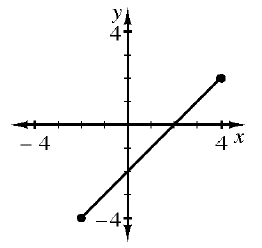b.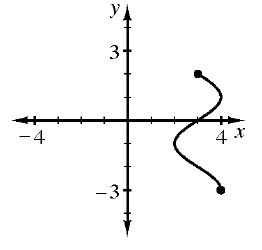c.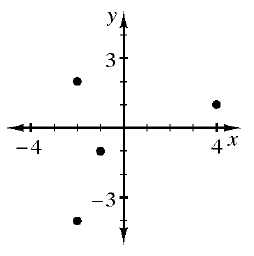d.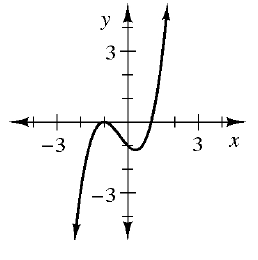e.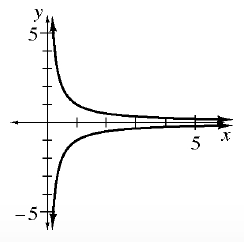f.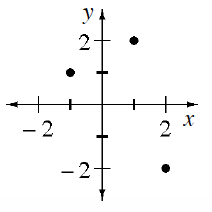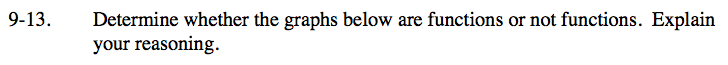A graph is only a function if there is no more than one y-value for any given x-value.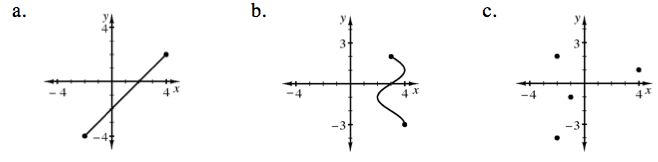Are there any points on this line segment that are above or below another point on the line segment? If there are, the graph is not a function.

This graph is a function.

See hint for part (a).

This graph is not a function. Some x-values are related to multiple y values. For example, when x is 3, y can be 2 or ≈ 2.

See hint for part (a).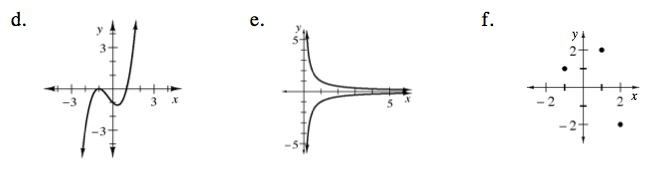See hint for part (a).

See hint for part (a).

This graph is not a function.

See hint for part (a).